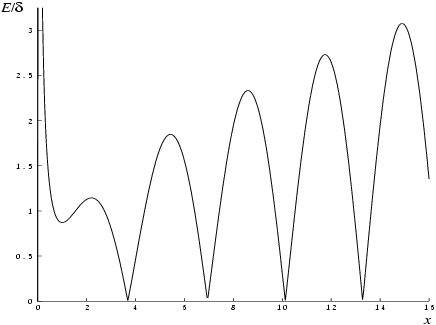NAG Library Function Document

1Purpose

nag_bessel_y1_vector (s17arc) returns an array of values of the Bessel function ${Y}_{1}\left(x\right)$.

2Specification

 #include #include
 void nag_bessel_y1_vector (Integer n, const double x[], double f[], Integer ivalid[], NagError *fail)

3Description

nag_bessel_y1_vector (s17arc) evaluates an approximation to the Bessel function of the second kind ${Y}_{1}\left({x}_{i}\right)$ for an array of arguments ${x}_{\mathit{i}}$, for $\mathit{i}=1,2,\dots ,n$.
Note:  ${Y}_{1}\left(x\right)$ is undefined for $x\le 0$ and the function will fail for such arguments.
The function is based on four Chebyshev expansions:
For $0,
 $Y1 x = 2π ln⁡x x8 ∑′r=0 ar Tr t - 2πx + x8 ∑′r=0 br Tr t , with ​ t = 2 x8 2 - 1 .$
For $x>8$,
 $Y1 x = 2πx P1 x sin x - 3 π4 + Q1 x cos x - 3 π4$
where ${P}_{1}\left(x\right)=\underset{r=0}{{\sum }^{\prime }}\phantom{\rule{0.25em}{0ex}}{c}_{r}{T}_{r}\left(t\right)$,
and ${Q}_{1}\left(x\right)=\frac{8}{x}\underset{r=0}{{\sum }^{\prime }}\phantom{\rule{0.25em}{0ex}}{d}_{r}{T}_{r}\left(t\right)$, with $t=2{\left(\frac{8}{x}\right)}^{2}-1$.
For $x$ near zero, ${Y}_{1}\left(x\right)\simeq -\frac{2}{\pi x}$. This approximation is used when $x$ is sufficiently small for the result to be correct to machine precision. For extremely small $x$, there is a danger of overflow in calculating $-\frac{2}{\pi x}$ and for such arguments the function will fail.
For very large $x$, it becomes impossible to provide results with any reasonable accuracy (see Section 7), hence the function fails. Such arguments contain insufficient information to determine the phase of oscillation of ${Y}_{1}\left(x\right)$; only the amplitude, $\sqrt{\frac{2}{\pi x}}$, can be determined and this is returned on failure. The range for which this occurs is roughly related to machine precision; the function will fail if  (see the Users' Note for your implementation for details).
Abramowitz M and Stegun I A (1972) Handbook of Mathematical Functions (3rd Edition) Dover Publications
Clenshaw C W (1962) Chebyshev Series for Mathematical Functions Mathematical tables HMSO

5Arguments

1:    $\mathbf{n}$IntegerInput
On entry: $n$, the number of points.
Constraint: ${\mathbf{n}}\ge 0$.
2:    $\mathbf{x}\left[{\mathbf{n}}\right]$const doubleInput
On entry: the argument ${x}_{\mathit{i}}$ of the function, for $\mathit{i}=1,2,\dots ,{\mathbf{n}}$.
Constraint: ${\mathbf{x}}\left[\mathit{i}-1\right]>0.0$, for $\mathit{i}=1,2,\dots ,{\mathbf{n}}$.
3:    $\mathbf{f}\left[{\mathbf{n}}\right]$doubleOutput
On exit: ${Y}_{1}\left({x}_{i}\right)$, the function values.
4:    $\mathbf{ivalid}\left[{\mathbf{n}}\right]$IntegerOutput
On exit: ${\mathbf{ivalid}}\left[\mathit{i}-1\right]$ contains the error code for ${x}_{\mathit{i}}$, for $\mathit{i}=1,2,\dots ,{\mathbf{n}}$.
${\mathbf{ivalid}}\left[i-1\right]=0$
No error.
${\mathbf{ivalid}}\left[i-1\right]=1$
 On entry, ${x}_{i}$ is too large. ${\mathbf{f}}\left[\mathit{i}-1\right]$ contains the amplitude of the ${Y}_{1}$ oscillation, $\sqrt{\frac{2}{\pi {x}_{i}}}$.
${\mathbf{ivalid}}\left[i-1\right]=2$
 On entry, ${x}_{i}\le 0.0$, ${Y}_{1}$ is undefined. ${\mathbf{f}}\left[\mathit{i}-1\right]$ contains $0.0$.
${\mathbf{ivalid}}\left[i-1\right]=3$
${x}_{i}$ is too close to zero, there is a danger of overflow. On failure, ${\mathbf{f}}\left[\mathit{i}-1\right]$ contains the value of ${Y}_{1}\left(x\right)$ at the smallest valid argument.
5:    $\mathbf{fail}$NagError *Input/Output
The NAG error argument (see Section 3.7 in How to Use the NAG Library and its Documentation).

6Error Indicators and Warnings

NE_ALLOC_FAIL
Dynamic memory allocation failed.
See Section 2.3.1.2 in How to Use the NAG Library and its Documentation for further information.
On entry, argument $〈\mathit{\text{value}}〉$ had an illegal value.
NE_INT
On entry, ${\mathbf{n}}=〈\mathit{\text{value}}〉$.
Constraint: ${\mathbf{n}}\ge 0$.
NE_INTERNAL_ERROR
An internal error has occurred in this function. Check the function call and any array sizes. If the call is correct then please contact NAG for assistance.
See Section 2.7.6 in How to Use the NAG Library and its Documentation for further information.
NE_NO_LICENCE
Your licence key may have expired or may not have been installed correctly.
See Section 2.7.5 in How to Use the NAG Library and its Documentation for further information.
NW_IVALID
On entry, at least one value of x was invalid.

7Accuracy

Let $\delta$ be the relative error in the argument and $E$ be the absolute error in the result. (Since ${Y}_{1}\left(x\right)$ oscillates about zero, absolute error and not relative error is significant, except for very small $x$.)
If $\delta$ is somewhat larger than the machine precision (e.g., if $\delta$ is due to data errors etc.), then $E$ and $\delta$ are approximately related by:
 $E ≃ x Y0 x - Y1 x δ$
(provided $E$ is also within machine bounds). Figure 1 displays the behaviour of the amplification factor $\left|x{Y}_{0}\left(x\right)-{Y}_{1}\left(x\right)\right|$.
However, if $\delta$ is of the same order as machine precision, then rounding errors could make $E$ slightly larger than the above relation predicts.
For very small $x$, absolute error becomes large, but the relative error in the result is of the same order as $\delta$.
For very large $x$, the above relation ceases to apply. In this region, ${Y}_{1}\left(x\right)\simeq \sqrt{\frac{2}{\pi x}}\mathrm{sin}\left(x-\frac{3\pi }{4}\right)$. The amplitude $\sqrt{\frac{2}{\pi x}}$ can be calculated with reasonable accuracy for all $x$, but $\mathrm{sin}\left(x-\frac{3\pi }{4}\right)$ cannot. If $x-\frac{3\pi }{4}$ is written as $2N\pi +\theta$ where $N$ is an integer and $0\le \theta <2\pi$, then $\mathrm{sin}\left(x-\frac{3\pi }{4}\right)$ is determined by $\theta$ only. If $x>{\delta }^{-1}$, $\theta$ cannot be determined with any accuracy at all. Thus if $x$ is greater than, or of the order of, the inverse of the machine precision, it is impossible to calculate the phase of ${Y}_{1}\left(x\right)$ and the function must fail.Figure 1

8Parallelism and Performance

nag_bessel_y1_vector (s17arc) is not threaded in any implementation.

None.

10Example

This example reads values of x from a file, evaluates the function at each value of ${x}_{i}$ and prints the results.

10.1Program Text

Program Text (s17arce.c)

10.2Program Data

Program Data (s17arce.d)

10.3Program Results

Program Results (s17arce.r)

© The Numerical Algorithms Group Ltd, Oxford, UK. 2017